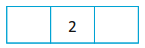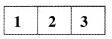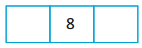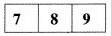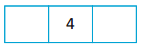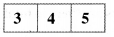# AP Board 1st Class Maths Solutions 1st Lesson Numbers (0-9)

Andhra Pradesh AP Board 1st Class Maths Solutions 1st Lesson Numbers (0-9) Textbook Exercise Questions and Answers.

## AP State Syllabus 1st Class Maths Solutions Chapter 1 Numbers (0-9)

Textbook Page No. 1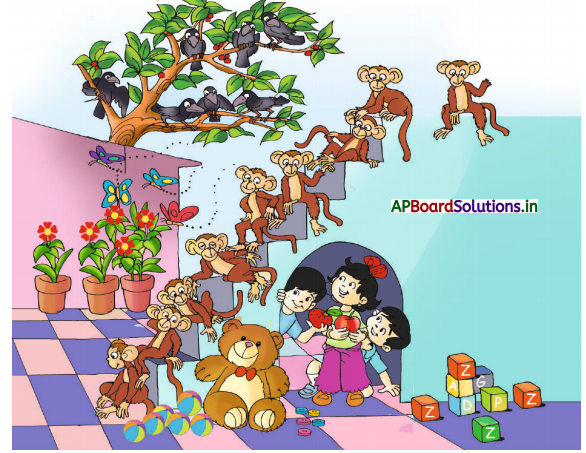Teacher note: Narrate the story. Get your pupils to observe and speak about the picture. Let them identify the things as per instructions.

Question 1.
What do you observe in the picture ?
There are butterflies, flower pots, monkeys, teddy bear, balls, tree, birds and three children.

Question 2.
What are the children doing.
They are seeing birds, monkeys and butterflies.Question 3.
Do you see the teddy bear ?
Yes

Question 4.
How many butterflies are there ?
4

Question 5.
How many boys are there ?
2

Question 6.
How many balls are there ?
6

Question 7.
How many birds are there ?
7

Question 8.
Are the leaves more than flowers ?
Yes

Question 9.
How many pots are there ?
3

Question 10.
Where are the monkeys ?
The monkeys are on the stairs.Textbook Page No. 2

One To One:
Match the following based on the number of objects.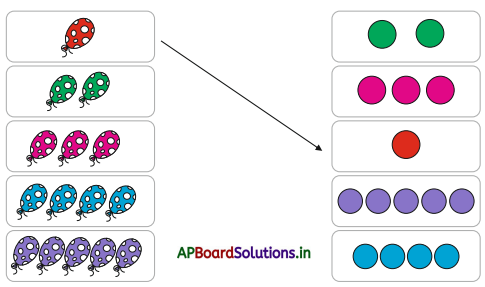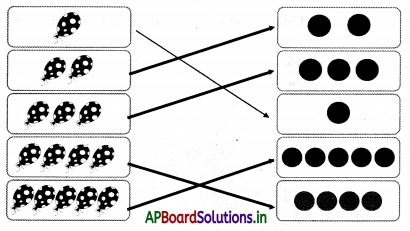Textbook Page No. 3

One – 1

Look at the picture given below. Count each thing. Tell the number of things of each type.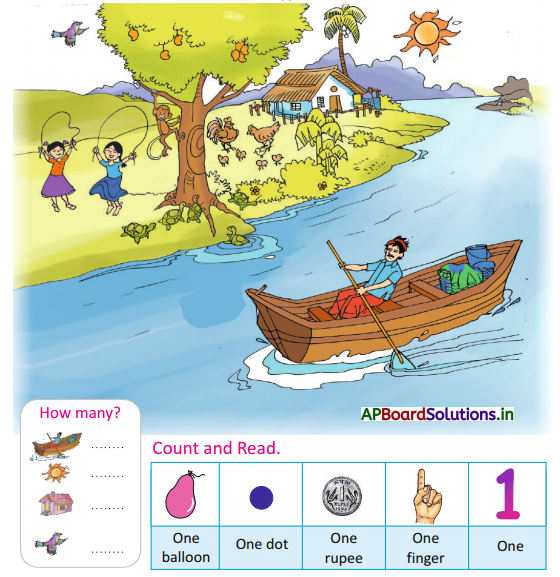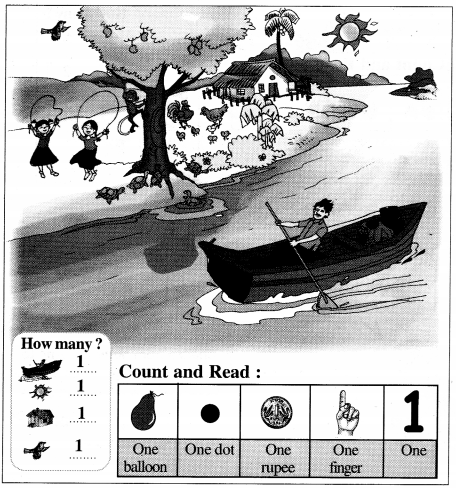Textbook Page No. 5

Two – 2

Look at the picture given below. Count each thing. Tell the number of things of each type.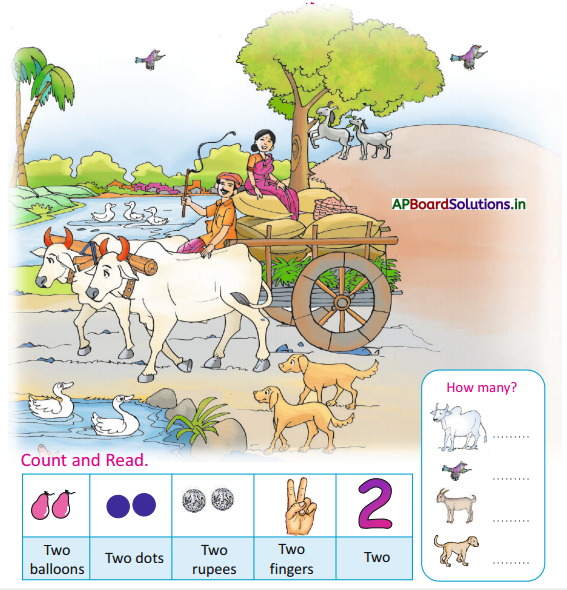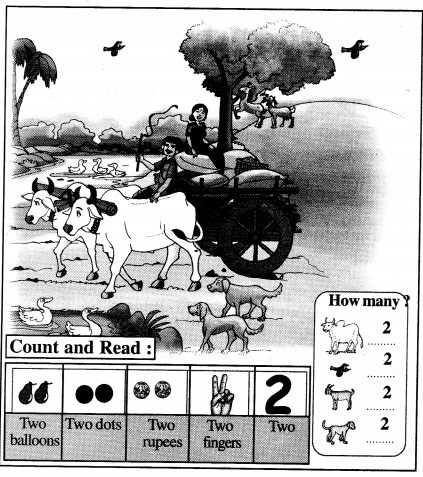Textbook Page No. 6

Look and Count: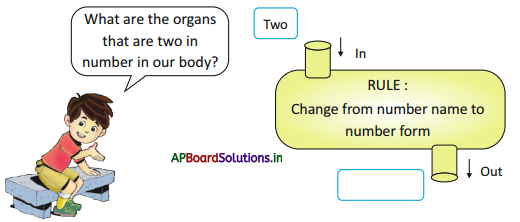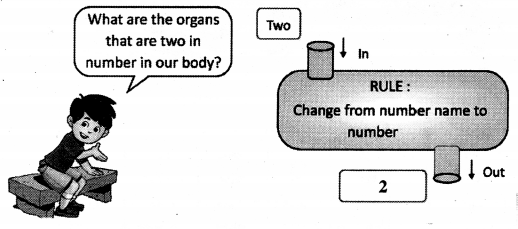Textbook Page No. 7

Three – 3

Look at the picture given below. Count each thing. Tell the number of things of each type.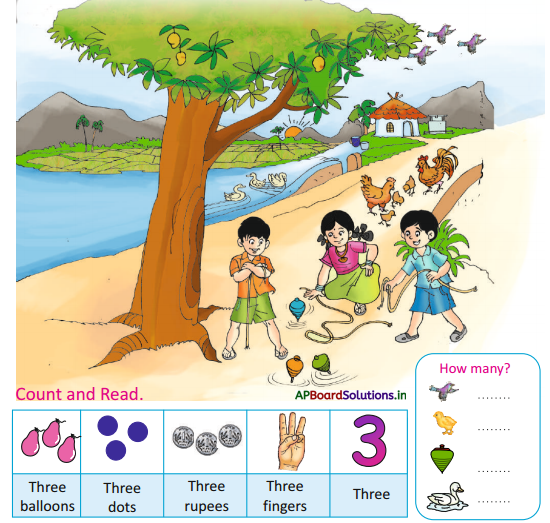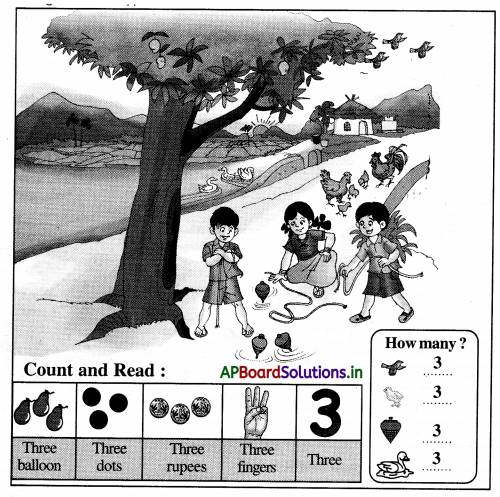Textbook Page No. 8

Look and Count: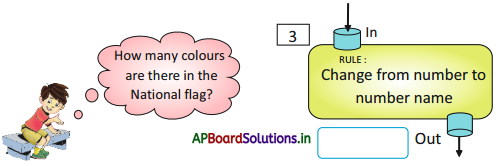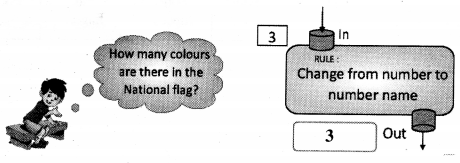Textbook Page No. 9

Four – 4

Look at the picture given below. Count each thing. Tell the number of things of each type.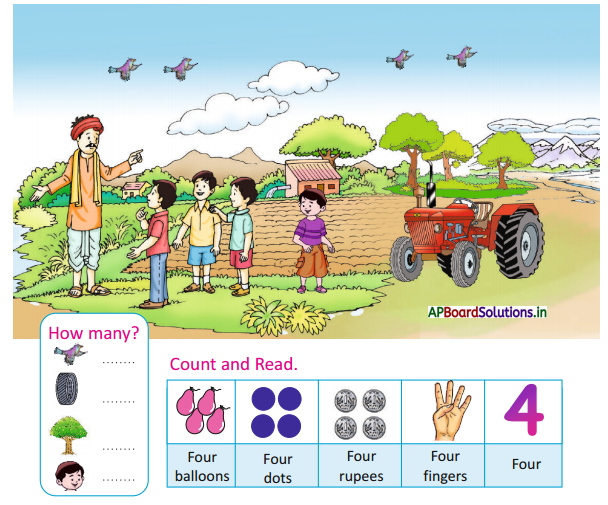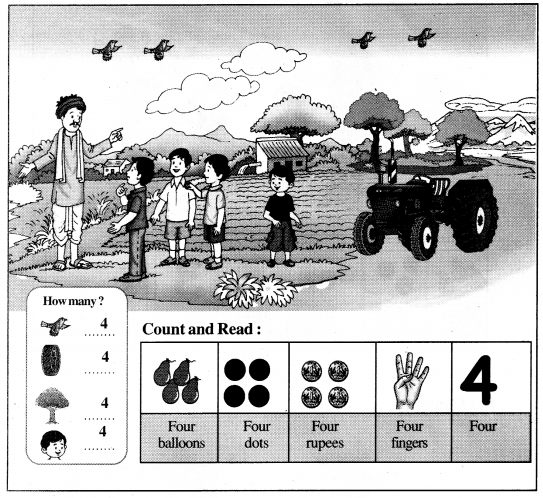Textbook Page No. 11

Five – 5

Look at the picture given below. Count each thing. Tell the number of things of each type.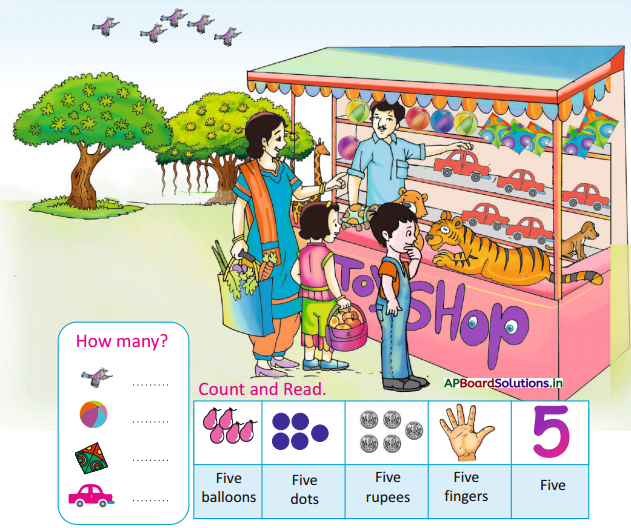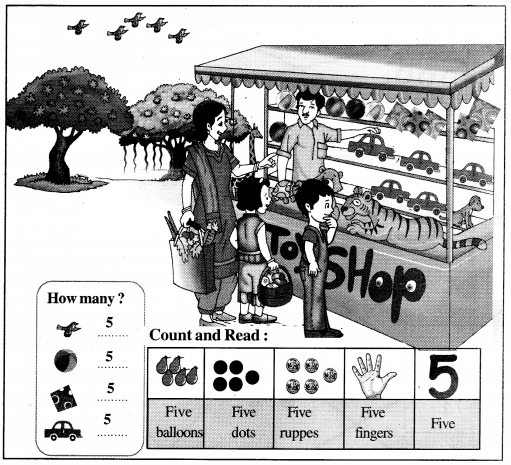Textbook Page No. 13

Match the following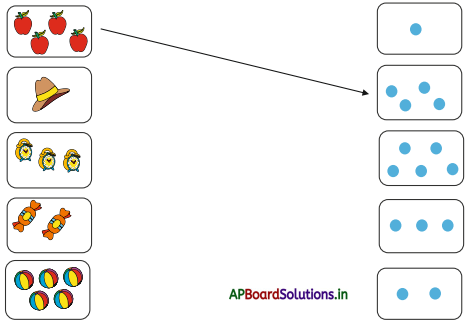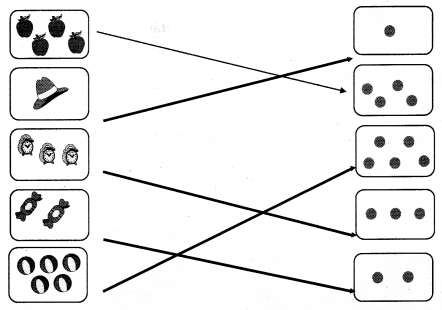Match the following :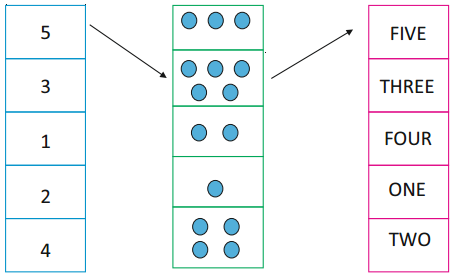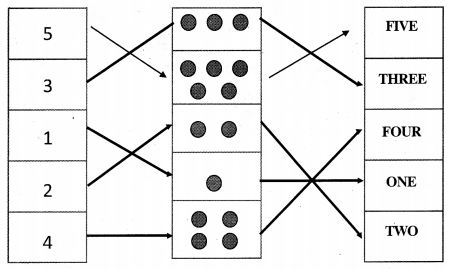Textbook Page No. 14

Numbers 6 To 9

Look at the picture given below. Count each thing. Tell the number of things of each type.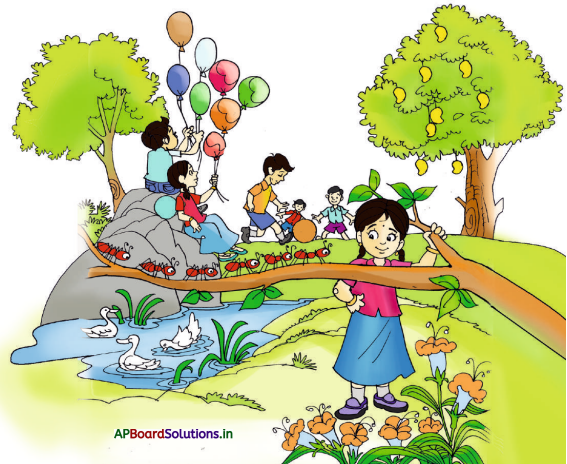Get your pupils to observe above picture.

Question 1.
What do you observe in the picture ?
Some children are playing. Mangoes, ants, ducks and some trees are in the picture. In the picture we observed 6 children, 8 balloons, 6 ants, 2 trees, 7 flowers and 3 ducks, 9 Mangoes are in the picture.

Question 2.
How many children are there ?
Six (6)Question 3.
How many mangoes are there ?
Nine (9)

Question 4.
How many ants are there ?
Six (6)

Textbook Page No. 16

Six-6

Look at the picture given below. Count each thing. Tell the number of things of each type.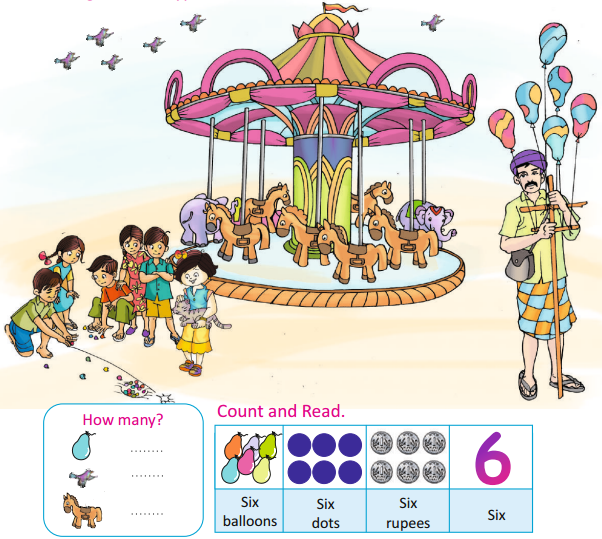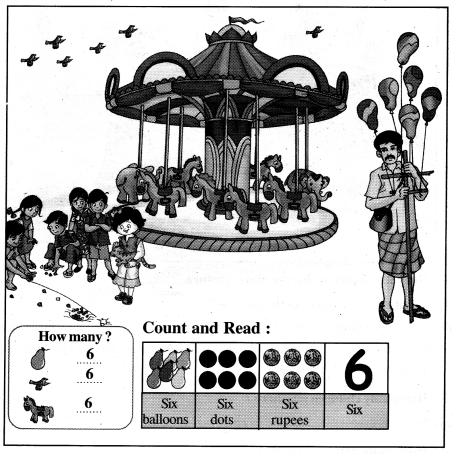Textbook Page No. 17

Seven-7

Look at the picture given below. Count each thing. Tell the number of things of each type.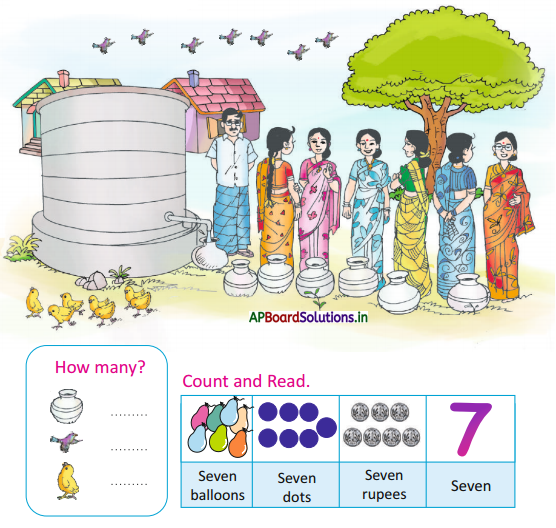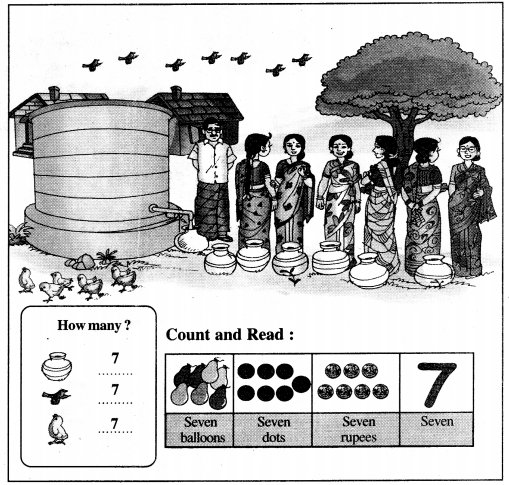Textbook Page No. 19

Eight – 8

Look at the picture given below. Count each thing. Tell the number of things of each type.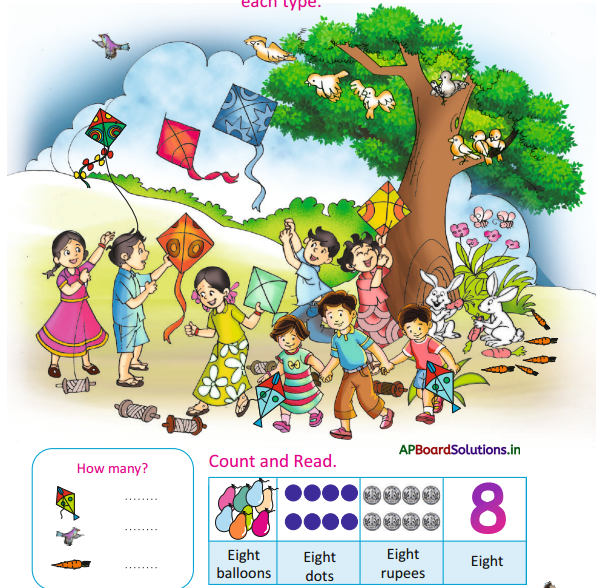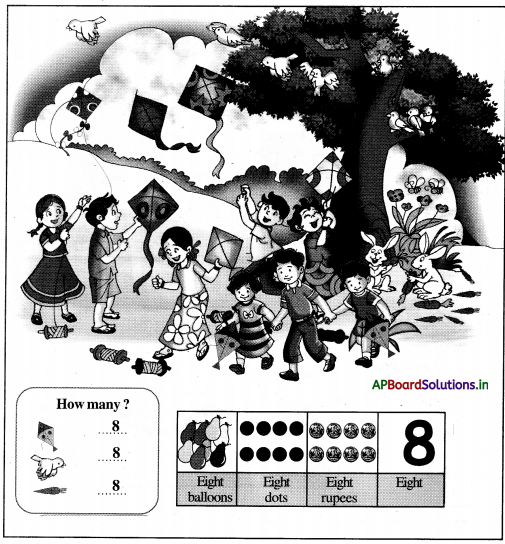Textbook Page No. 21

Nine – 9

Look at the picture given below. Count each thing. Tell the number of things of each type.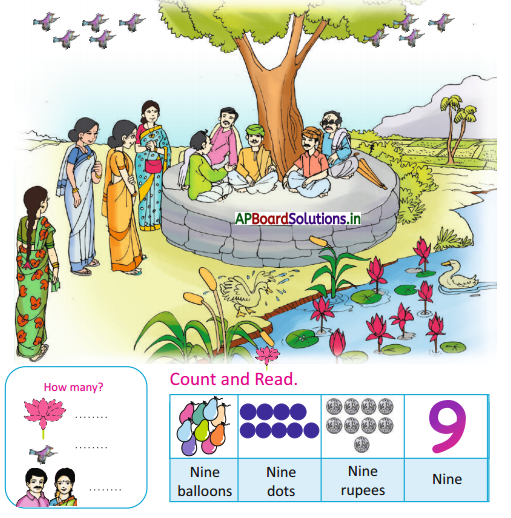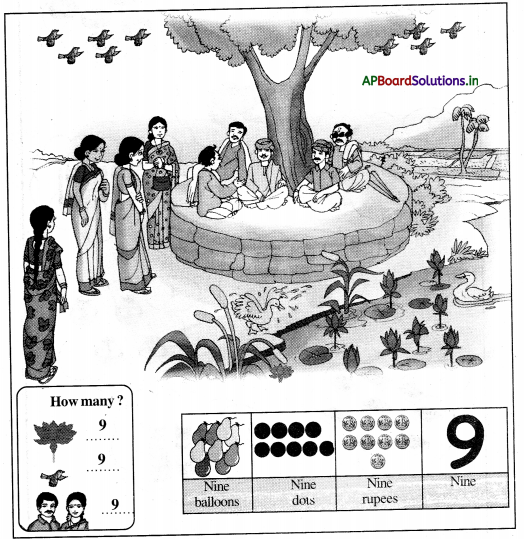Textbook Page No. 23

Count the objects and write the correct number in the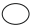.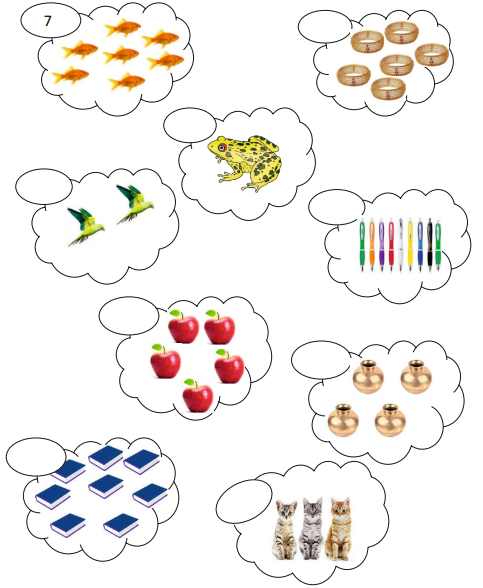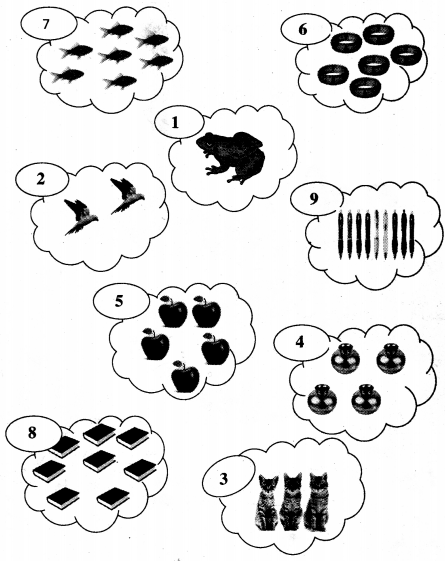Textbook Page No. 24

Circle the correct number.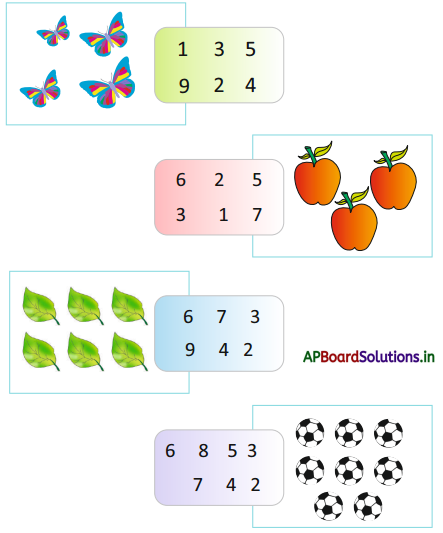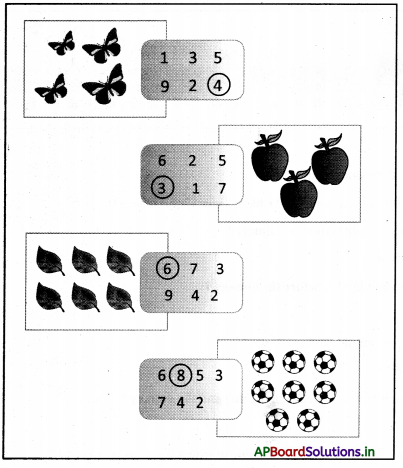Textbook Page No. 26

Bujji is counting the titles in a row. You also count each colour of the tile. Tell the number of black colour and white colour tiles in each row.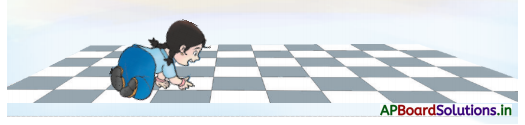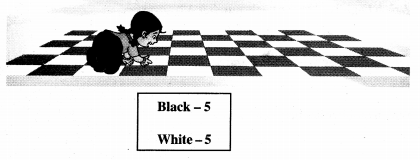Textbook Page No. 27

Before – Between – After :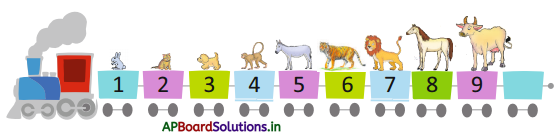Question 1.
Tell the names of animals.
Rabbit, cat, dog, monkey, Donkey, Tiger, Lion, Horse, Ox.

Question 2.
What is your favourite animals ?
Monkey

Question 3.
Which animal is before the donkey ?
Monkey

Question 4.
Which animal is after the donkey ?
Tiger

Question 5.
Which animal is between the dog and the donkey ?
MonkeyQuestion 6.
What is the number of the bogie in which the donkey is found ?
5

Question 7.
Which bogie is before the bogie number 4 ?
3

Question 8.
Which bogie is after the bogie number 4 ?
5

Question 9.
Tell the number in between 6 and 8.
7

Question 10.
Which number lies between 7 and 9 ?
8

Textbook Page No. 28

Try this:

Circle the number before 6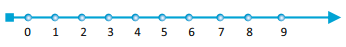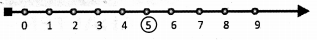Circle the number before 5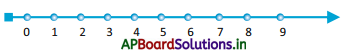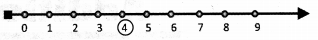Circle the number after 3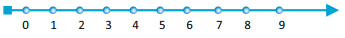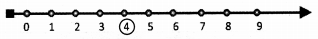Circle the number after 7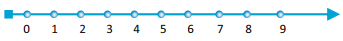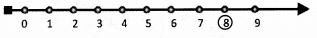Textbook Page No. 29

Exercise

What comes before and after.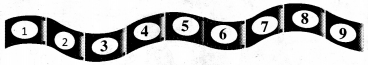Write the numbers from 9 to 1.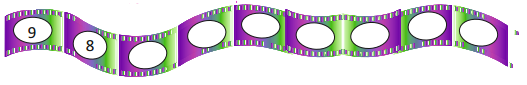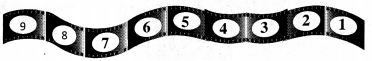Write the number that comes after.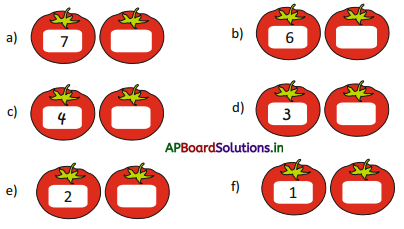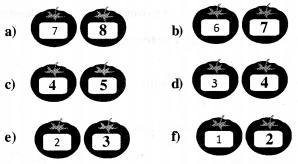What comes before and after.

a)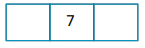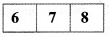b)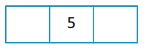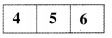c)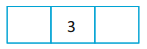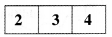d)